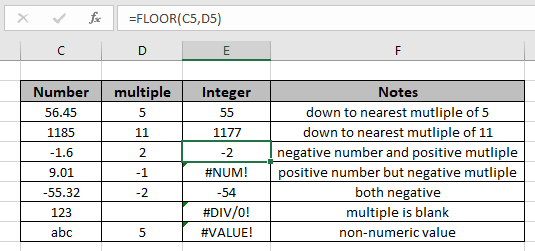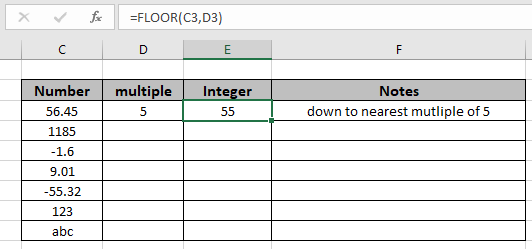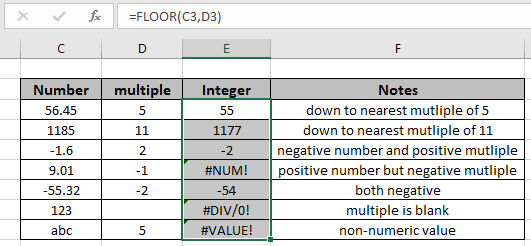# How to use the FLOOR function in ExcelIn this article, we will learn about how to use the FLOOR function in Excel. The FLOOR function rounds down the number to the nearest specified multiple. FLOOR rounds to the nearest integer, using a 1 as significance. Positive numbers with decimal values are rounded down to the nearest integer (e.g. 6.7 is rounded down to 6) while negative numbers with decimal values are rounded away from zero (e.g. -5.8 is rounded to -6).

The FLOOR function rounds down a number to the nearest multiple.
Syntax:

=FLOOR (number, significance)

number : number to be rounded off

significance : multiple to use when rounding

Example:

All of these might be confusing to understand. So, let's test this formula via running it on the example shown below. Here we will perform the FLOOR function over different numbers and significance with the numbers.

Use the formula:

=FLOOR(C3,D3)

Explanation:
1. The nearest number in the multiple of 5 (significance) with respect to 56.45 (number).
2. The returned number must be less than the input numberAs you can see in the above snapshot that the function returns 55 which is the nearest integer in the multiple of 5. Now to apply the formula to the remaining cell just copy the formula using Ctrl + D taking the first cell till the last cell needed cell formula.As you can see, the function rounds down the number to a specified multiple.

Here are some observational notes shown below.

Notes:

1. The formula only works with numbers.
2. The formula returns the nearest last multiple given input any decimal number.
3. Negative significance is only allowed when number itself is negative else the formula returns #NUM! error.
4. Blank cell or 0 as significance value returns #DIV/0! error.
5. The function returns #VALUE! Error if the date or time value is invalid.

Hope this article about How to use the FLOOR function in Excel is explanatory. Find more articles on mathematical formulas here. If you liked our blogs, share it with your fristarts on Facebook. And also you can follow us on Twitter and Facebook. We would love to hear from you, do let us know how we can improve, complement or innovate our work and make it better for you. Write to us at info@exceltip.com.

Related Articles

How to use the ROUND function in Excel | Rounds off the given number to the nearest num_digit decimal using the ROUND function in Excel

How to use the ROUNDUP function in Excel | Rounds up the given number to the nearest num_digit decimal using the ROUNDUP function in Excel

How to use the ROUNDDOWN function in Excel | Rounds down the given number to the nearest num_digit decimal using the ROUNDDOWN function in Excel

How to use the FLOOR.MATH function in Excel | rounds down the number to the nearest specified multiple using the FLOOR.MATH function in Excel

How to use the MROUND function in Excel | rounds off the number to the nearest specified multiple using the MROUND function in Excel.

Popular Articles:

50 Excel Shortcuts to Increase Your Productivity | Get faster at your task. These 50 shortcuts will make you work ODD faster on Excel.

The VLOOKUP Function in Excel | This is one of the most used and popular functions of excel that is used to lookup value from different ranges and sheets.

COUNTIF in Excel 2016 | Count values with conditions using this amazing function. You don't need to filter your data to count specific values. Countif function is essential to prepare your dashboard.

How to Use SUMIF Function in Excel | This is another dashboard essential function. This helps you sum up values on specific conditions.

Terms and Conditions of use

The applications/code on this site are distributed as is and without warranties or liability. In no event shall the owner of the copyrights, or the authors of the applications/code be liable for any loss of profit, any problems or any damage resulting from the use or evaluation of the applications/code.